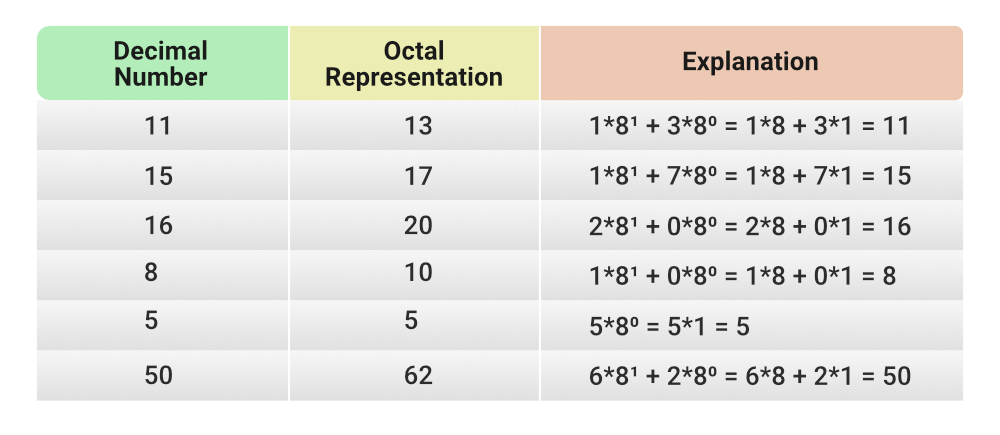# Java Program to Illustrate the usage of Octal Integer

• Last Updated : 22 Jun, 2021

Octal is a number system where a number is represented in powers of 8. So all the integers can be represented as an octal number. Also, all the digit in an octal number is between 0 and 7. In java, we can store octal numbers by just adding 0 while initializing. They are called Octal Literals. The data type used for storing is int.The method used to convert Decimal to Octal is Integer.toOctalString(int num)

Syntax:

`public static String toOctalString(int num)`

Parameters: The method accepts a single parameter num of integer type which is required to be converted to a string.

Return Value: The function returns a string representation of the integer argument as an unsigned integer in base 8.

Example 1

## Java

 `import` `java.io.*;` `class` `GFG {``    ``public` `static` `void` `main(String[] args)``    ``{``        ``// Variable Declaration``        ``int` `a;` `        ``// storing normal integer value``        ``a = ``20``;``        ``System.out.println(``"Value of a: "` `+ a);` `        ``// storing octal integer value``        ``// just add 0 followed octal representation``        ``a = ``024``;``        ``System.out.println(``"Value of a: "` `+ a);` `        ``// convert octal representation to integer``        ``String s = ``"024"``;``        ``int` `c = Integer.parseInt(s, ``8``);``        ``System.out.println(``"Value of c: "` `+ a);` `        ``// get octal representation of a number``        ``int` `b = ``50``;``        ``System.out.println(``            ``"Octal Representation of the number "` `+ b``            ``+ ``" is: "` `+ Integer.toOctalString(b));``    ``}``}`

Output
```Value of a: 20
Value of a: 20
Value of c: 20
Octal Representation of the number 50 is: 62```

Example 2: The different arithmetic operation can also be performed on this octal integer. Operation is the same as performed on the int data type.

## Java

 `// Arithmetic operations on Octal numbers` `import` `java.io.*;` `class` `GFG {``    ``public` `static` `void` `main(String[] args)``    ``{``        ``int` `a, b;` `        ``// 100``        ``a = ``0144``;` `        ``// 20``        ``b = ``024``;` `        ``System.out.println(``"Value of a: "` `+ a);``        ``System.out.println(``"Value of b: "` `+ b);``        ``System.out.println(``"Addition: "` `+ (a + b));``        ``System.out.println(``"Subtraction: "` `+ (a - b));``        ``System.out.println(``"Multiplication: "` `+ (a * b));``        ``System.out.println(``"Division: "` `+ (a / b));``    ``}``}`

Output
```Value of a: 100
Value of b: 20